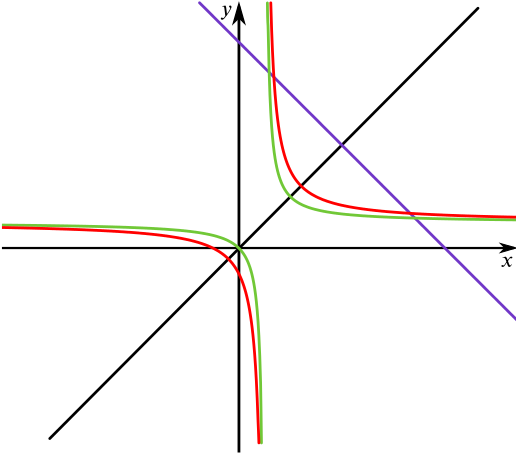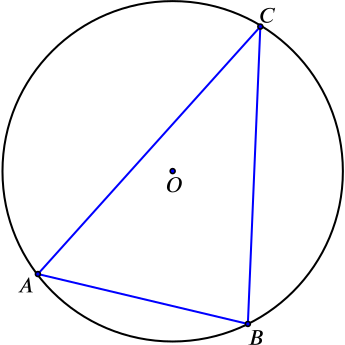# Symmetry

Symmetry is something we all understand instinctively; it seems to be hardwired into our brains. As mathematical concepts go, symmetry is perhaps the one that people find easiest to relate to. But what exactly is symmetry and where in mathematics can we find it? If you haven’t thought about this before, the answer may not be that obvious: symmetry is invariance under change.

A shape is symmetric if it remains the same when we apply a certain operation to it. In the case of shapes drawn on the plane the operations we are interested in are those that move a shape without changing distances between points. We have already met three types of such rigid motions: reflections, rotations and translations (moving an object without rotating or distorting it) which can be seen in Which parabola?. And this is almost all there is. In two dimensions there is one other type of symmetry describing a line of footprints in the sand. In this case the picture remains the same when we translate it by a certain amount and then reflect it, this type of rigid motion is called a glide reflection.

We usually think of symmetry as something we can see; something associated with patterns and shapes. But the more general concept of symmetry—invariance under change—means that all sorts of other objects can be symmetric too, including mathematical expressions. Think for example of the expression $7x^6-10x^4+4x^2-1$. It’s a higher degree polynomial than we normally see, but because all the powers involved are even, its value is the same for both $x$ and $-x$, a transformation $x\mapsto -x$.

If you would like to explore these ideas in more detail then you start with That’s odd … or even and look at some families of functions in Odd or even or….

Being able to spot symmetries in equations is extremely useful. For example, we often want to find the values of $x$ for which an expression $f(x)$ is equal to zero. The symmetries in $f(x)=f(-x)$ means that once we have found such a value, its negative also gives us an answer to $f(x)=0$. This suggests a problem-solving principle, in that if we know that a problem has a symmetric solution then that solution can be easier to find. Just as with the example above, the symmetry also means that when sketching the graph of the function $f(x)$ we only need to work out its shape for non-negative values of $x$ and the rest follows, or vice versa. The graph, which is again a visual object, is symmetric under reflection in the $y$-axis. This reflection can often make solving equations of the form $\cos x = \dots$ much easier than $\sin x=\dots$.

When curves have other lines of symmetry this can tell you something very particular about the function that gives rise to the curve. What symmetries can be found in Name that graph or in Composing gets me nowhere and what algebraic properties do they correspond to? Once you have identified a symmetry for a curve you can then exploit it to solve problems involving them, like in Problem areas.From Composing gets me nowhere

Problems too can contain symmetries. Rewriting an expression in a certain form can reveal a different type of symmetry, one that can be explored to make solving a problem easier such as in the Review questions How do we deal with an equation that includes a square root? and Given two simultaneous equations of degree two, can we solve them?.

Seeing the symmetry in a problem can also give you an insight into how the problem was created, such as in Scary sum. It can also make the task of finding solutions easier. In A difference of two fractions symmetry can reduce the problem to looking at a smaller set of expressions.

Symmetry may also offer insight into a bigger idea which lies behind a mathematical object. In Irrational roots knowing how the symmetry of the intersections of a parabola with the $x$-axis relates to the roots of a quadratic equation $ax^2+bx+c=0$, with $a$, $b$ and $c$ rational numbers, gives rise to a general method of finding roots for quadratic equations.

Seeing symmetry as invariance under change gives rise to invariants for the mathematical object or the mathematical problem we are looking at. Such as in Sine-ing the way which explores a connection between the sine of the angles and the side lengths of a triangle, during which an invariant for the triangle is discovered.From Sine-ing the way

Symmetry is one of the ideas that we have chosen to highlight in our pervasive ideas.